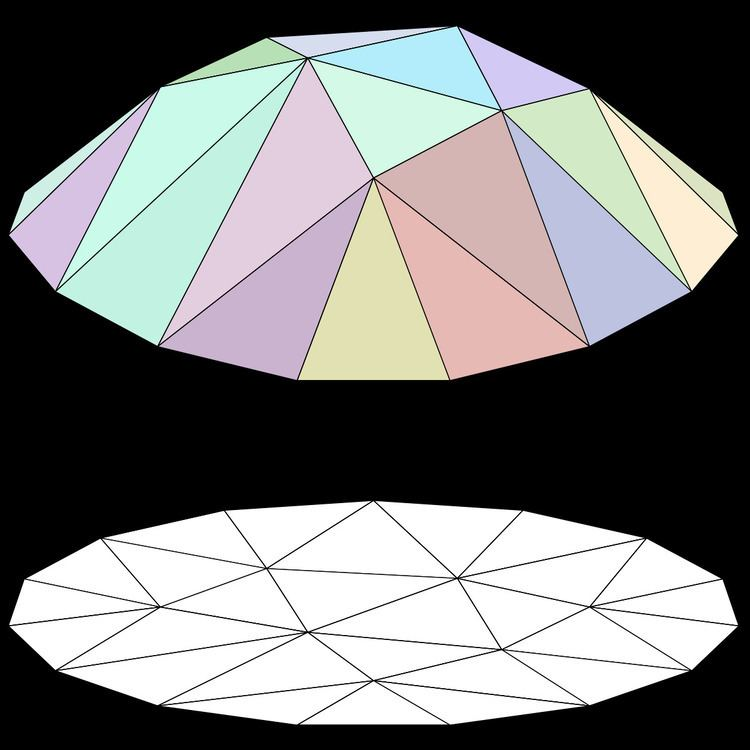# Polyhedral terrain

Updated on
Covid-19In computational geometry, a polyhedral terrain in three-dimensional Euclidean space is a polyhedral surface that intersects every line parallel to some particular line in a connected set (i.e., a point or a line segment) or the empty set. Without loss of generality, we may assume that the line in question is the z-axis of the Cartesian coordinate system. Then a polyhedral terrain is the image of a piecewise-linear function in x and y variables.

## Contents

The polyhedral terrain is a generalization of the two-dimensional geometric object, the monotone polygonal chain.

As the name may suggest, a major application area of polyhedral terrains include geographic information systems to model real-world terrains.

## Representation

A polyhedral model may be represented in terms of the partition of the plane into polygonal regions, each region being associated with a plane patch which is the image of points of the region under the piecewise-linear function in question.

## Problems

There a number of problems in computational geometry which involve polyhedral terrains.

## References

Similar Topics
Honeymoon in Vegas
Thierry Steimetz
Kang Susie
Topics